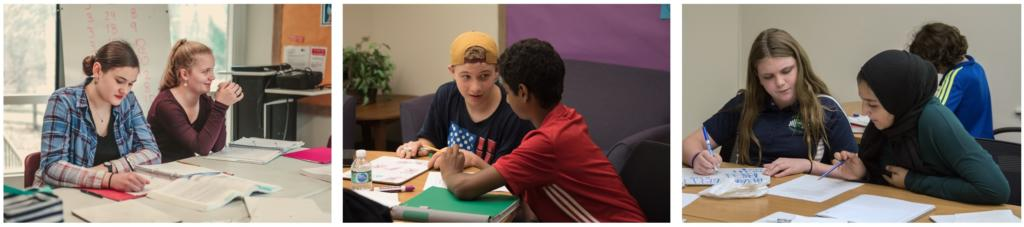# NEW: Math Lab at Compass

## One way to make sure the math work is getting done…

Introducing Math Lab at Compass! Math Lab is a tutoring center where students can go for weekly help on math homework! Math Lab begins on Wednesday, September 25. An experienced Compass math teacher will provide on-the-spot help with math homework for any middle school math, Pre-Algebra, or Algebra I.

In Math Lab, homeschooled students bring the math homework they are assigned- whether from a Compass math class, an online math program, or material taught at home. Sometimes just having a helper nearby or hearing a math explanation from someone else will make the concept “click.”

How does Math Lab work?

• Bring a current math assignment including any textbook, workbook, or worksheets
• Work independently until you have a question, reach a stumbling block, or need clarification on a concept or computation.
• An experienced Compass math instructor will be circling the room and continually checking in with students.
• The tutor will help students check answers, remind them of a technique, or demonstrate a different way to solve the problem.
• A partial list of topics that students can receive help on includes: number lines, integers, negative numbers, fractions, decimals, percentages, factoring, exponents, roots, order of operations, inequalities, coordinate plane, working with variables, solving equations, and word problems.

Students can sign up to attend Math Lab at 9:00 am (with math teacher Natalie DiVietri) or at 3:00 pm (with math teacher David Chelf). Current registration is for a 5-week session in Quarter 1, beginning on September 25.

Register for Math Lab online. The cost of Math Lab is \$99.00 for the five sessions, a fraction of the price of a math tutor (\$60-\$90 per hour!). The price of Math Lab is further discounted to \$79.00 for students in Compass’s year-long Pre-Algebra or Algebra classes. Math Lab will continue to be offered in future sessions by quarter. Math Lab students can purchase a discounted subscription to IXL Math, on online learning platform for more math help.

Would you like to see tutors for other levels of math? Contact Compass know if you have a student who would be interested in elementary math, higher levels of high school math (geometry, algebra II, etc), or something else.